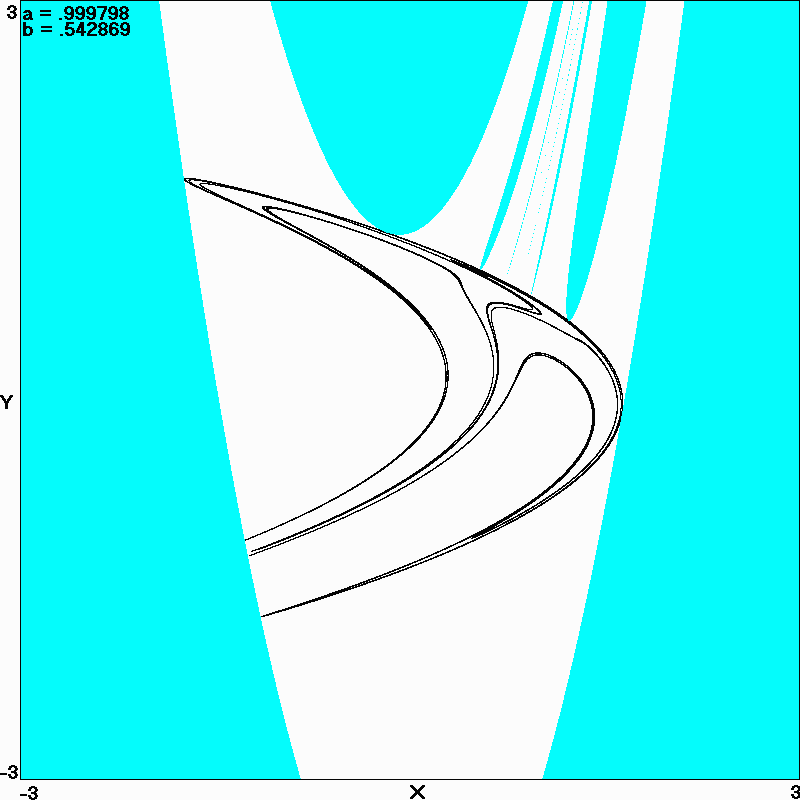# Henon Map Maximum Lyapunov Exponent and Kaplan-Yorke Dimension

J. C. Sprott
Department of Physics, University of Wisconsin, Madison, WI 53706, USA
March 13, 2007

The Henon map is a prototypical 2-D invertible dissipative iterated map with chaotic solutions proposed by the French astronomer Michel Henon (M. Henon, Commun. Math. Phys. Phys. 50, 69-77 (1976)) as a simplified model of the Poincare map for the Lorenz model:
xn+1 = 1 - axn2 + byn
yn+1 = xn

Since the second equation above can be written as yn = xn-1, the Henon map can be written in terms of a single variable with two time delays:
xn+1 = 1 - axn2 + bxn-1

An interesting question to ask is what are the values of a and b that maximize the largest Lyapunov exponent and the Kaplan-Yorke dimension. The maximum Lyapunov exponent occurs for a = 2 and b = 0, where the map reduces to a one-dimensional quadratic map whose largest Lyapunov exponent is ln(2) = 0.693147181... . The other exponent is minus infinity, which implies that there is infinitely rapid contraction in the direction perpendicular to the one-dimensional parabolic attractor.

Much less trivial is the question of what values of a and b maximize the Kaplan-Yorke dimension. The area contraction for the Henon map is the sum of the two Lyapunov exponents and is given by ln(|b|) which is negative (contractive) for -1 < b < 1 and encompasses the entire region over which the map is bounded as well as chaotic. From the largest Lyapunov exponent L, the Kaplan-Yorke dimension is therefore given by Dky = 1 + L/(L - ln|b|).

A program was written to search ab-plane for the maximum value of Dky giving a result that is suspiciously close to a = 1.0 with b = 0.5427 for which L = 0.2716 and Dky = 1.3076. The attractor for this case along with its basin of attraction (in white) is shown below:Note that the attractor nearly touches its basin boundary in numerous places, which is typical for a chaotic system where the maximum chaos often occurs just before the orbit becomes unbounded. Note also that the basin boundary has numerous (perhaps infinitely many) narrow tongues, suggesting a possibly fractal structure that deserves further study. The attractor is not greatly different from the Henon map with the usual parameters of a = 1.4 and b = 0.3 for which L = 0.419222 and Dky = 1.258269 (see Sprott). Thus the usual parameters are close to those for which the attractor dimension is maximized, and all of the attractors are distinctly "Henon-like."

Back to Sprott's Technical Notes